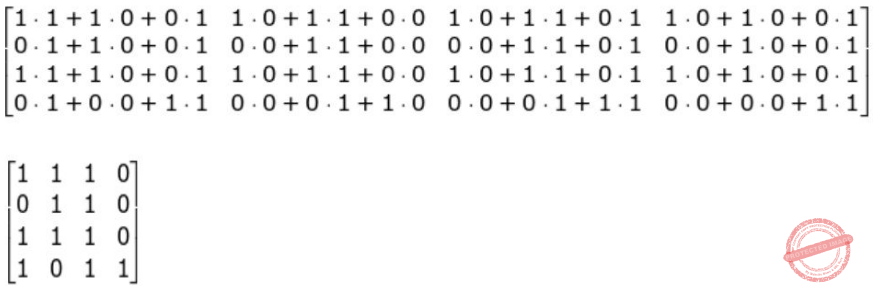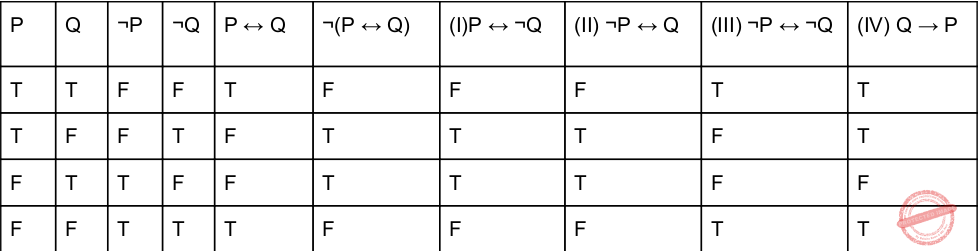## UGC NET CS 2017 Nov- paper-2

 Question 1
If the time is now 4 O’clock, what will be the time after 101 hours from now ?
 A 9 O’Clock B 8 O'Clock C 5 O’Clock D 4 O’Clock
genereal-aptitude       Time-clock
Question 1 Explanation:
Per day 24 hours.
Present time given 4 O’clock.
Method-1: After 24+24+24+24=96. it means after 96 hours again time is 4 O’clock.
Remaining 5 hours. So, total 4+5=9 O’Clock
Method-2: Perform modulus operation by 24. So, 101%24=5
= Given time + modules time
= 4+5=9 O’Clock
 Question 2Find the boolean product A⊙B of the two matrices.
 ABCDEngineering-Mathematics       Linear-Algebra
Question 2 Explanation:
In this problem, they given boolean product A⊙B of two matrices.
The boolean product truth table isAccording to the truth table, we have to perform matrix multiplication.Question 3
How many distinguishable permutations of the letters in the word BANANA are there ?
 A 720 B 120 C 60 D 360
Engineering-Mathematics       Probability
Question 3 Explanation:
Total distinguishable permutations means no repetition.
BANANA= 6 letters
B is appearing 1 time
A is appearing 3 times
N is appearing 2 times
So, = 6! / (3! * 2!)
= 60
 Question 4
The Octal equivalent of the binary number 1011101011 is :
 A 7353 B 1353 C 5651 D 5657
Digital-Logic-Design       Number-Systems
Question 4 Explanation:
We have to divide binary number into 3 bit pairs from LSB.
1 011 101 011
1 3 5 3
(1011101011)​ 2​ = (1353)​ 8
 Question 5
Let P and Q be two propositions, ¬ (P ↔ Q) is equivalent to:
(I) P ↔ ¬ Q
(II) ¬ P ↔ Q
(III) ¬ P ↔ ¬ Q
(IV) Q → P
 A Only (I) and (II) B Only (II) and (III) C Only (III) and (IV) D None of the above
Engineering-Mathematics       Propositional-Logic
Question 5 Explanation:So, (I) and (Ii) are TRUE.
 Question 6
‘ptrdata’ is a pointer to a data type. The expression *ptrdata++ is evaluated as (in C++) :
 A *(ptrdata++) B (*ptrdata)++ C * (ptrdata) ++ D Depends on compiler
Programming-in-c++       Data-types
Question 6 Explanation:
Normally ++ having higher precedence than * but both are unary operators in C++.
So, the precedence ptrdata++ will evaluate first. So, we are given parenthesis (ptrdata++).
Second precedence is * and finally *(ptrdata++)
 Question 7
The associativity of which of the following operators is Left to Right, in C++ ?
 A Unary Operator B Logical not C Array element access D addressof
Programming-in-c++       Operators
Question 7 Explanation:Note: The array element access evaluates from left to right and starts index 0.
 Question 8
A member function can always access the data in __________ , (in C++).
 A the class of which it is member B the object of which it is member C the public part of its class D the private part of its class
Programming-in-c++       Functions
Question 8 Explanation:
→ A member function can always access the data in the class of which it is member.
→ Functions within classes can access and modify (unless the function is constant) data members without declaring them, because the data members are already declared in the class.
 Question 9
Which of the following is not correct for virtual function in C++ ?
 A Must be declared in public section of class B Virtual function can be static C Virtual function should be accessed using pointers. D Virtual function is defined in base class
Programming-in-c++       Virtual-Functions
Question 9 Explanation:
→ Virtual functions are called only for objects of class types, you cannot declare global or static functions as virtual.
 Question 10
Which of the following is not correct (in C++) ?
 A Class templates and function templates are instantiated in the same way B Class templates differ from function templates in the way they are initiated C Class template is initiated by defining an object using the template argument D Class templates are generally used for storage classes E None of the above
Programming-in-c++       Functions
Question 10 Explanation:
(1) TRUE: Class templates and function templates are instantiated in the same way
(2) FALSE: Class templates differ from function templates in the way they are initiated
(3) FALSE: Class template is initiated by defining an object using the template argument
(4)FALSE: Class templates are generally used for storage classes
 Question 11
Which of the following is/are true with reference to ‘view’ in DBMS ?
(a) A ‘view’ is a special stored procedure executed when certain event occurs.
(b) A ‘view’ is a virtual table, which occurs after executing a pre-compiled query. code:
 A Only (a) is true B Only (b) is true C Both (a) and (b) are true D Neither (a) nor (b) are true
Database-Management-System       SQL
Question 11 Explanation:
VIEW is a virtual table based on the result set of a SQL statement.
→ In SQL, a view is a virtual table based on the result-set of an SQL statement.
→ A view contains rows and columns, just like a real table. The fields in a view are fields from one or more real tables in the database.
→ You can add SQL functions, WHERE, and JOIN statements to a view and present the data as if the data were coming from one single table.
CREATE VIEW Syntax:
CREATE VIEW view_name AS
SELECT column1, column2, ...
FROM table_name
WHERE condition;
Note: A view always shows up-to-date data! The database engine recreates the data, using the view's SQL statement, every time a user queries a view.
 Question 12
In SQL, __________ is an Aggregate function.
 A SELECT B CREATE C AVG D MODIFY
Database-Management-System       SQL
Question 12 Explanation:
Aggregate function is AVG. The AVG() function returns the average value of a numeric column.
There are 12 questions to complete.

Register Now